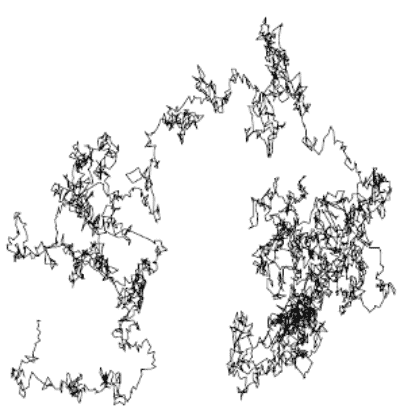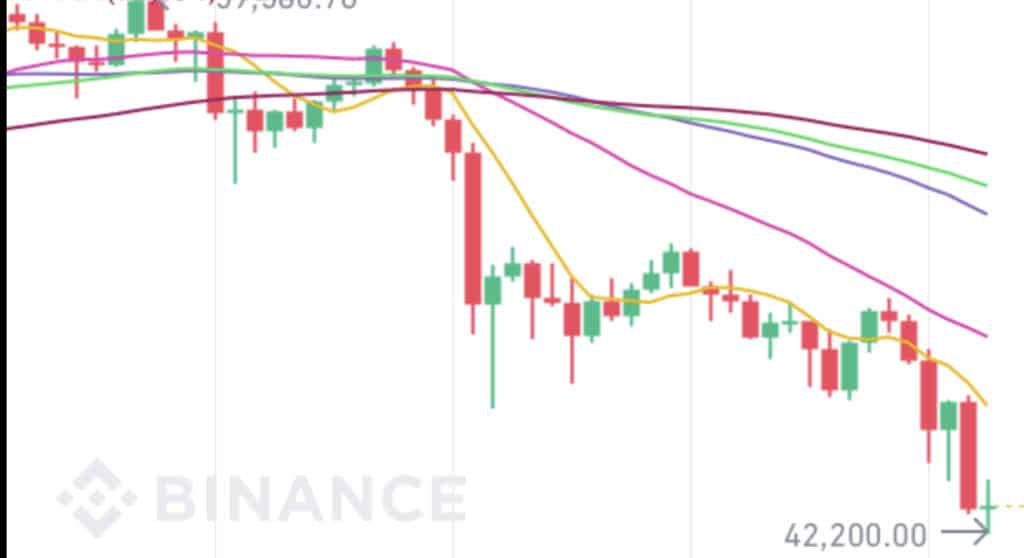Wiener process

We start considering a symmetric random walker, moving along a 1-dimensional lattice, with lattice constant $a$. When the random walker is located $m$ sites away from the origin, its position will be $x=a m$. The walker hops at discrete times $t=n \tau, n=0,1,2 \ldots$ with equal probability to the right or to the left
$$P(m, t+\tau \mid m-1, t)=\frac{1}{2}, \quad P(m, t+\tau \mid m+1, t)=\frac{1}{2}$$
We will consider the limit where the jump size $a$ and the time step $\tau$ are both small. Using the Markov property $P(n, t+\tau)=\sum_{m} P(m, t) P(n, t+\tau \mid m, t)$,
$$P(m, t+\tau)=\frac{1}{2} P(m-1, t)+\frac{1}{2} P(m+1, t)$$
Subtracting equal quantities on RHS and LHS
$$P(m, t+\tau)-P(m, t)=\frac{1}{2}[P(m-1, t)+P(m+1, t)-2 P(m, t)]$$
and rewriting in terms of $x$, we get
\begin{aligned} P(x, t+\tau)-P(x, t) &=\frac{1}{2}[P(x-a, t)+P(x+a, t)-2 P(x, t)] \ &=\frac{1}{2}[P(x-a, t)-P(x, t)]+\frac{1}{2}[P(x+a, t)-P(x, t)] \end{aligned}
Taylor expanding for small $a$ and $\tau$
$$\tau \frac{\partial P(x, t)}{\partial t}=\frac{1}{2}\left[-a \frac{\partial P(x, t)}{\partial x}+\frac{a^{2}}{2} \frac{\partial^{2} P(x, t)}{\partial x^{2}}+a \frac{\partial P(x, t)}{\partial x}+\frac{a^{2}}{2} \frac{\partial^{2} P(x, t)}{\partial x^{2}}\right]$$Bitcoin短期内的的价格波动是一个Wiener过程

and eventually taking the limits $a \rightarrow 0$ and $\tau \rightarrow 0$, in such a way that $a^{2} / \tau=2 D$ is constant, leads to the famous diffusion equation
$\frac{\partial P(x, t)}{\partial t}=D \frac{\partial^{2} P(x, t)}{\partial x^{2}}$
describing the motion of a Brownian particle immersed in a fluid (see next section for more detail). The continuous-time stochastic process $x(t)$ obeying the probabilistic law given by the solution of the diffusion equation, is known as the Wiener process.

Equation (5.3) is also known as the heat equation, as it had been originally derived to describe how the distribution of heat evolves over time in a solid medium, as it spontaneously flows from places where it is higher towards places where it is lower (since $D>0, P(x, t)$ is an increasing function of time when it is convex and decreasing when it is concave). Over the years, it has been used in a wide variety of disciplines, for example, in financial mathematics, it is applied to the paradigmatic Black-Scholes model for pricing.随机游走代写认准UpriviateTA

BS equation代写